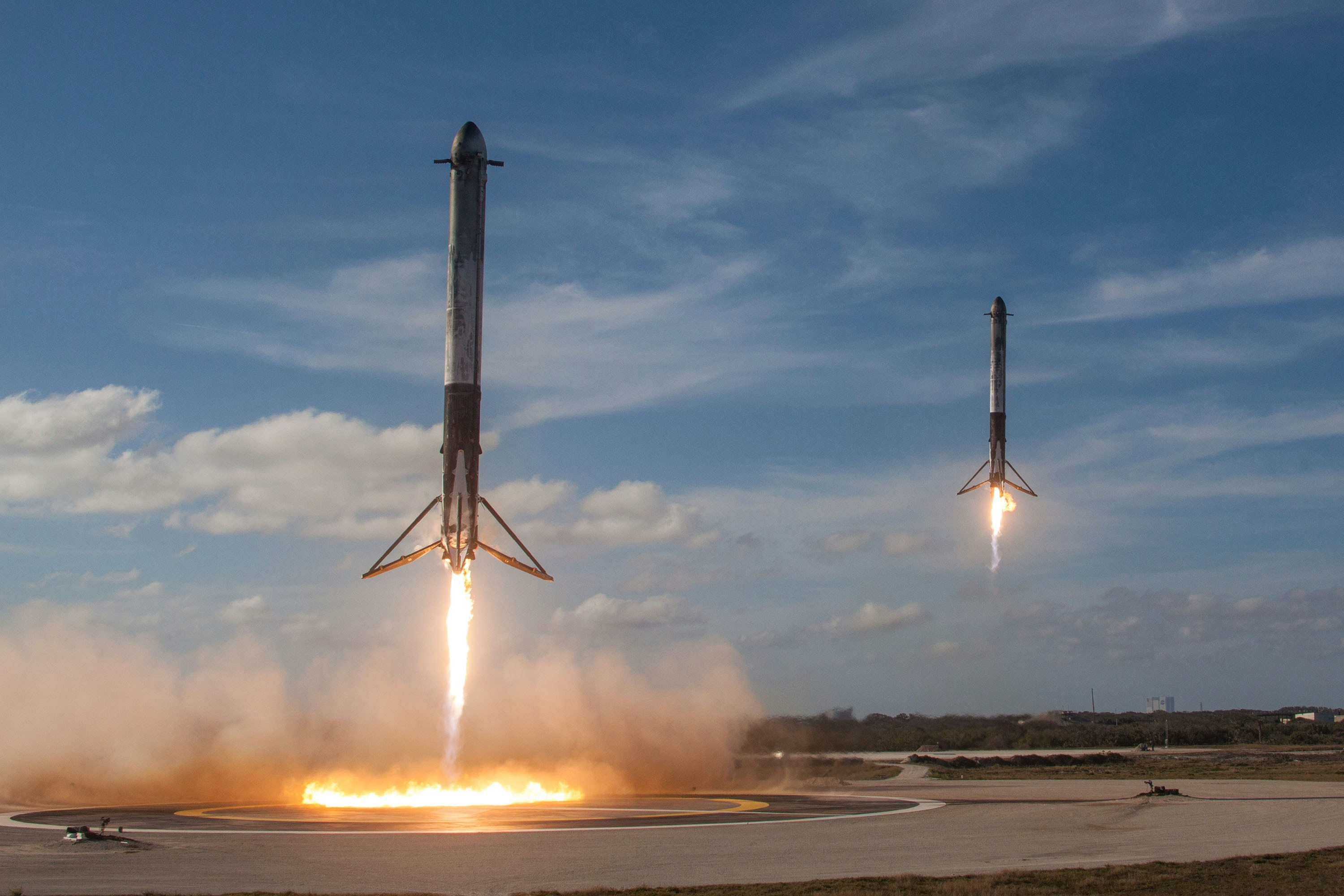# Rocket Science : Landing On The Moon

Rocket Science : Landing On The Moon

Goal & Method

We are attempting to plot the trajectory of a rocket on a mission to land on the Moon.

We neglect the effect of the Earth’s atmosphere or the changing mass of the rocket as it burns its fuel or loses its booster rockets. The only variables we will need to worry about are the initial velocity of the rocket and the launch angle relative to a chosen reference frame. To simplify calculations, we propose the moon has mass “1” and radius “1” and change our gravitational constant accordingly.

#### The Physics

The theoretical equation of motion can be derived by dividing m on both sides.

Since the law of gravitation states that acceleration is inversely proportional to distance squared, the differential equation is not an easy one to solve.

We therefore employed a numerical method developed by Euler to obtain an accurate approximation. We choose a time step and evolve the system assuming the time step is so small that the integrand is constant.

The exercise is separated into 2 sections.

In the first, we use Euler’s method to calculate the trajectory of the rocket using Euler’s method for a stationary moon. In the second, we use the improved Euler’s method to calculate the trajectory of the rocket for a moon moving in a circular orbit around the earth. The moon is at (0,222) when t=0.

Results

Figure 1. Output of the first section with 10s time step and launching angle 104.6 degrees

Figure 2. Output from second section with 1s time step and launching angle 104.6 degrees

Figure 3. Output from second section with 10s time step and launching angle 104.6 degrees

Figure 4. Output from second section with 0.1s time step

Figure 5. Output from second section with 0.1s time step and launching angle 104.4 degrees

Conclusions

Time step doesn’t affect the result by a lot in the first section since the COM of moon, earth, and trajectory lies in a straight line. In the second section, however, we can see that the change in time step (0.1s, 1s, and 10s) affects the outcome as the rocket lands on the moon only when we set a time step equal to 1. This is mainly because gravitational acceleration is not aligned with initial velocity and this affects the direction of the rocket by a lot in the initial stages.

If we use delta t=0.1s, the rocket lands on the moon if we change the launching angle to 104.4 degrees.

Appendix: my Code

Euler’s method with stationary moon

% Author: Runlai Xu, Wadham College , Date: 02/12/2021

% Define initial parameters

init_pos = [0, 3.7];

init_vel = 0.0066 * [cosd(89.9), sind(89.9)];

moon_pos = [0, 222];

% Set time step to be 10s

t = linspace(0, 300000, 30000);

simulate_rocket(init_pos, init_vel, moon_pos, t)

[tout, pos] = simulate_rocket(init_pos, init_vel, moon_pos, t);

% Plot final result

scatter(pos(:,1), pos(:,2));

hold

plot(0,222,’r*’)

function [tout, pos] = simulate_rocket(init_pos, init_vel, moon_pos, t)

% Simulate the rocket trajectory with the Earth and Moon influence. The coordinate

% used in this function is centred at Earth‚Äôs centre (i.e. Earth centre at (0,0) )

% Input:

% * init_pos:

% * init_vel:

% * moon_pos:

% The simulation finishes when it simulates for the whole t, or the rocket landed

% on the Moon.

% * t: an N‚àíelements vector of the time step where the position of the rocket will be % returned. 15 %

% Output:

% * tout: an N‚àíelements array where the position is described % * pos: (M x 2) matrix indicating the positions of the rocket as function of time, column is x and the second column is y.

% initialize v and constants

tout = t;

pos = zeros(30000,2);

vel = zeros(30000,2);

vel(1,:)= init_vel;

pos(1,:)= init_pos;

moon=1;

earth=83.3;

G=9.63*10^-7;

for i=2:100000

% calculate new position

pos(i,:)= pos(i-1,:)+vel(i-1,:)*10;

% calculate acceleration due to the moon and earth

accE=-G*(earth*pos(i-1,:)/((pos(i-1,1))^2+(pos(i-1,2))^2)^1.5); acc=accE+G*moon*(moon_pos-pos(i-1,:))/((pos(i-1,1))^2+(moon_pos(2)- pos(i-1,2))^2)^1.5;

%calculate new velocity

vel(i,:)=vel(i-1,:)+acc*10;

if ((pos(i,1))^2+(moon_pos(2)-pos(i,2))^2)^0.5<1

% check if the rocket lands on the moon

break

end

end

end

Improved Euler’s method with revolving moon

% Author: Runlai Xu, Wadham College , Date: 02/12/2021

% Define initial parameters

init_pos = [0, 3.7];

init_vel = 0.0066 * [cosd(104.4), sind(104.4)];

moon_pos = [0, 222];

% Set time step to be 0.1s

t = linspace(0, 300000, 3000000);

% Call function and give output

simulate_rocket(init_pos, init_vel, moon_pos, t)

[tout, pos] = simulate_rocket(init_pos, init_vel, moon_pos, t);

% Plot final result

scatter(pos(:,1), pos(:,2));

function [tout, pos] = simulate_rocket(init_pos, init_vel, moon_pos, t)

% Simulate the rocket trajectory with the Earth and Moon influence. The coordinate

% used in this function is centred at Earth‚Äôs centre (i.e. Earth centre at (0,0) )

% Input:

% * init_pos:

% * init_vel:

% * moon_pos:

% The simulation finishes when it simulates for the whole t, or the rocket landed

% on the Moon.

% * t: an N‚àíelements vector of the time step where the position of the rocket will be % returned. 15 %

% Output:

% * tout: an N‚àíelements array where the position is described % * pos: (M x 2) matrix indicating the positions of the rocket as function of time, column is x and the second column is y.

% initialize variables and constants

tout = t;

pos = zeros(3000000,2);

vel = zeros(3000000,2);

vel(1,:)= init_vel;

pos(1,:)= init_pos;

moon=1;

earth=83.3;

G=9.63*10^-7;

Ro=222;

w=2.6615*10^-6;

for i=2:3000000

% calculate moon position at time t

moonPos = @(t) Ro*[cos(w*t+pi/2), sin(w*t+pi/2)];

moon_pos=moonPos(t(i));

% calculate estimated new position

pos(i,:)= pos(i-1,:)+vel(i-1,:)*0.1;

% calculate acceleration based on old position

accE1=-G*(earth*pos(i-1,:)/((pos(i-1,1))^2+(pos(i-1,2))^2)^1.5); acc1=accE1+G*moon*(moon_pos-pos(i-1,:))/((moon_pos(1)-pos(i 1,1))^2+(moon_pos(2)-pos(i-1,2))^2)^1.5;

% calculate acceleration based on estimated new position accE2=-G*(earth*pos(i-1,:)/((pos(i-1,1))^2+(pos(i-1,2))^2)^1.5); acc2=accE2+G*moon*(moon_pos-pos(i,:))/((moon_pos(1)-

pos(i,1))^2+(moon_pos(2)-pos(i,2))^2)^1.5;

% calculate new velocity by taking the integral of average acceleration vel(i,:)=vel(i-1,:)+(acc1+acc2)*0.05;

% calculate new position by taking the integral of average velocity pos(i,:)= pos(i-1,:)+(vel(i,:)+vel(i-1,:))*0.05;

if ((moon_pos(1)-pos(i,1))^2+(moon_pos(2)-pos(i,2))^2)^0.5<1 break

end

end

end

Rocket Science : Landing On The Moon Written by Runlai XuRocket Science : Landing On The Moon Written by Runlai Xu

Rocket Science